Sinusoidal Amplitude Modulation (AM) Next  |  Prev  |  Up  |  Top  |  Index  |  JOS Index  |  JOS Pubs  |  JOS Home  |  Search

### Sinusoidal Amplitude Modulation (AM)

It is instructive to study the modulation of one sinusoid by another. In this section, we will look at sinusoidal Amplitude Modulation (AM). The general AM formula is given by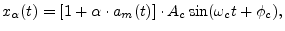where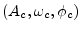are parameters of the sinusoidal carrier wave,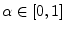is called the modulation index (or AM index), and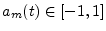is the amplitude modulation signal. In AM radio broadcasts,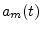is the audio signal being transmitted (usually bandlimited to less than 10 kHz), and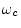is the channel center frequency that one dials up on a radio receiver. The modulated signal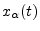can be written as the sum of the unmodulated carrier wave plus the product of the carrier wave and the modulating wave: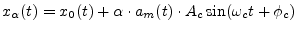(4.1)

In the case of sinusoidal AM, we have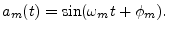(4.2)

Periodic amplitude modulation of this nature is often called the tremolo effect when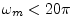or so (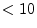Hz).

Let's analyze the second term of Eq. (4.1) for the case of sinusoidal AM with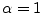and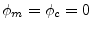: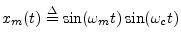(4.3)

An example waveform is shown in Fig.4.11 for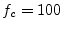Hz and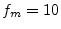Hz. Such a signal may be produced on an analog synthesizer by feeding two differently tuned sinusoids to a ring modulator, which is simply a four-quadrant multiplier'' for analog signals.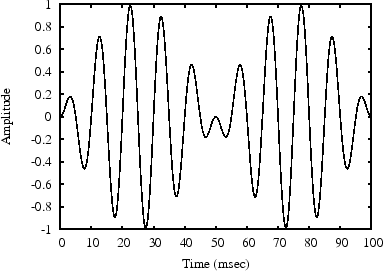When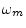is small (say less than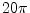radians per second, or 10 Hz), the signal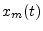is heard as a beating sine wave'' with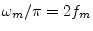beats per second. The beat rate is twice the modulation frequency because both the positive and negative peaks of the modulating sinusoid cause an amplitude swell'' in. (One period of modulation--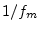seconds--is shown in Fig.4.11.) The sign inversion during the negative peaks is not normally audible.

Recall the trigonometric identity for a sum of angles: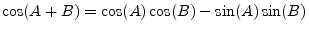Subtracting this from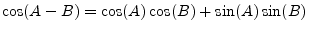leads to the identity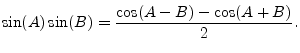Setting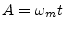and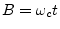gives us an alternate form for our ring-modulator output signal'':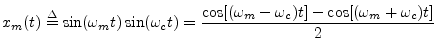(4.4)

These two sinusoidal components at the sum and difference frequencies of the modulator and carrier are called side bands of the carrier wave at frequency(since typically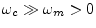).

Equation (4.3) expressesas a beating sinusoid'', while Eq. (4.4) expresses as it two unmodulated sinusoids at frequencies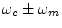. Which case do we hear?

It turns out we hearas two separate tones (Eq. (4.4)) whenever the side bands are resolved by the ear. As mentioned in §4.1.2, the ear performs a short time Fourier analysis'' of incoming sound (the basilar membrane in the cochlea acts as a mechanical filter bank). The resolution of this filterbank--its ability to discern two separate spectral peaks for two sinusoids closely spaced in frequency--is determined by the critical bandwidth of hearing [44,74,85]. A critical bandwidth is roughly 15-20% of the band's center-frequency, over most of the audio range . Thus, the side bands in sinusoidal AM are heard as separate tones when they are both in the audio range and separated by at least one critical bandwidth. When they are well inside the same critical band, beating'' is heard. In between these extremes, near separation by a critical-band, the sensation is often described as roughness'' .

Subsections
Next  |  Prev  |  Up  |  Top  |  Index  |  JOS Index  |  JOS Pubs  |  JOS Home  |  Search

[How to cite this work] [Order a printed hardcopy]